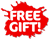ISBN: 978-1-988548-44-9

## Level 3 Statistics Externals Learning Workbook (new edition)ESA Publications

• 2695

Level 3 Statistics Externals Learning Workbook covers the three externally assessed NCEA Level 3 Mathematics and Statistics Achievement Standards:

• AS 91584 (Mathematics and Statistics 3.12): Evaluate statistically based reports
• AS 91585 (Mathematics and Statistics 3.13): Apply probability concepts in solving problems
• AS 91586 (Mathematics and Statistics 3.14): Apply probability distributions in solving problems
Each of these standards is worth 4 credits. The workbook features:
• concise theory notes with brief, clear explanations
• worked examples with key concepts and skills explained
• relevant sets of exercises for student practice
• answers to all exercises, provided in the back of the book
• revision and practice assessment tasks.

An index gives easy access to important terms and definitions highlighted in the text.

This workbook provides a variety of problems for the student. Basic questions require fundamental concepts to be applied in order to analyse statistical reports or to solve straightforward probability problems. More challenging problems require interpretation of the more complex aspects of a report, such as sources of variability or bias; or the calculation of probabilities involving multiple skills, such as the solving of inverse probability problems, or the selection and justification of a probability distribution to model a situation. The write-on format of this workbook keeps notes and exercises together in a compact form, providing a useful exam revision tool.

This workbook is ideal for use in the classroom, or for homework, home study and revision.

Published: 11/2021